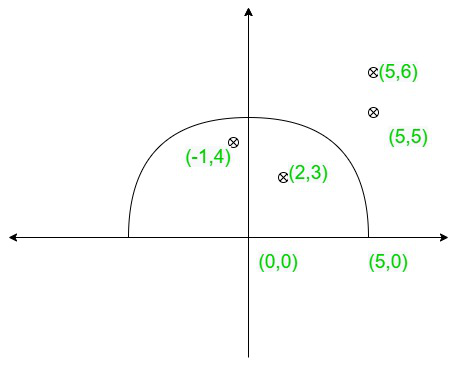Count points from an array that lies inside a semi-circle

• Last Updated : 28 May, 2021

Given two pairs (X, Y), (P, Q) and R the coordinate of the center of semi-circle, coordinate of the intersection of semicircle and diameter of the semicircle and, the radius of the semicircle, and an array arr[] of dimension N*2 consisting of the coordinates of few points, the task is to find the number of points from the array that lies inside or on the
semicircle
Note: The semicircle above the diameter is considered.

Examples:

Input: X = 0, Y = 0, R = 5, P = 5, Q = 0, arr[][] = { {2, 3}, {5, 6}, {-1, 4}, {5, 5} }
Output: 2
Explanation: The points {2, 3} and {-1, 4} are inside the semi-circle.Input: X = 2, Y = 3, R = 10, P = 12, Q = 3, arr[][] = { {-7, -5}, {0, 6}, {11, 4} }
Output: 2

Approach: The given problem can be solved based on the following observations:

• The points that lies on or inside the semicircle must be above or on the diameter of semicircle and the distance between center and that point should be ≤ R.
• Supposeis the equation of diameter.
The point (R, S) lies above the line if• A point (R, S) lies above the line formed by joining points (X, Y) and (P, Q) ifFollow the steps below to solve the problem:

• Find the equation of line the diameter of the semi-circle from the points (X, Y) and (P, Q).
• Initialize a variable, say ans, to store the count of required points.
• Traverse the array arr[] and perform the following operations:
• Calculate the distance between the points (X, Y) and (P, Q) and store it in a variable, say d.
• Put arr[i] and arr[i] in the place of R and S respectively, in the formulaand store the result in a variable, say f.
• Increment the count of ans by 1 if R ≤ d and f ≥ 0.
• After completing the above steps, print the value stored in ans.

Below is the implementation of the above approach:

C++

 // C++ program for above approach#include using namespace std; int getPointsIns(int x1, int y1, int radius, int x2,                 int y2, vector> points){    int ans = 0;         // Traverse the array    for(int i = 0; i < points.size(); i++)    {                 // Stores if a point lies        // above the diameter or not        bool condOne = false, condTwo = false;        if ((points[i].second - y2) *              (x2 - x1) - (y2 - y1) *             (points[i].first - x2) >= 0)        {            condOne = true;        }         // Stores if the R is less than or        // equal to the distance between        // center and point        if (radius >= (int)sqrt(pow((y1 - points[i].second), 2) +                                 pow(x1 - points[i].first, 2)))        {            condTwo = true;        }        if (condOne && condTwo)        {            ans += 1;        }    }    return ans;} // Driver codeint main(){    int X = 0;    int Y = 0;    int R = 5;    int P = 5;    int Q = 0;     vector> arr = { make_pair(2, 3),                                   make_pair(5, 6),                                   make_pair(-1, 4),                                   make_pair(5, 5) };     cout << getPointsIns(X, Y, R, P, Q, arr);    return 0;} // This code is contributed by nirajgusain5

Java

 // Java program for above approachimport java.io.*; class Gfg {  public static int getPointsIns(int x1, int y1,int radius,                                 int x2,int y2, pair points[])  {    int ans = 0;    // Traverse the array    for (int i = 0; i < points.length; i++)    {             // Stores if a point lies      // above the diameter or not      boolean condOne = false, condTwo = false;      if ((points[i].b - y2) *          (x2 - x1)- (y2 - y1) *          (points[i].a - x2)>= 0)      {        condOne = true;      }       // Stores if the R is less than or      // equal to the distance between      // center and point      if (radius >= (int)Math.sqrt(Math.pow((y1 - points[i].b), 2)+                                   Math.pow(x1 - points[i].a, 2)))      {        condTwo = true;      }      if (condOne && condTwo)      {        ans += 1;      }    }    return ans;  }     // Driver code  public static void main(String[] args)  {    int X = 0;    int Y = 0;    int R = 5;    int P = 5;    int Q = 0;     pair arr[] = {new pair(2, 3), new pair(5, 6), new pair(-1, 4), new pair(5,5)};     System.out.print(getPointsIns(X, Y, R, P, Q, arr));  }}class pair{  int a;  int b;  pair(int a,int b)  {       this.a = a;    this.b = b;  }}

Python3

 # Python implementation of above approachdef getPointsIns(x1, y1, radius, x2, y2, points):    # Stores the count of ans    ans = 0     # Traverse the array    for point in points:         # Stores if a point lies        # above the diameter or not        condOne = (point - y2) * (x2 - x1) \                  - (y2 - y1) * (point - x2) >= 0         # Stores if the R is less than or        # equal to the distance between        # center and point         condTwo = radius >= ((y1 - point) ** 2 \                  + (x1 - point) ** 2) ** (0.5)         if condOne and condTwo:            ans += 1     return ans  # Driver Code# InputX = 0Y = 0R = 5P = 5Q = 0arr = [[2, 3], [5, 6], [-1, 4], [5, 5]] print(getPointsIns(X, Y, R, P, Q, arr))

C#

 // C# program for above approachusing System; class Gfg{  public static int getPointsIns(int x1, int y1,                                 int radius, int x2,                                 int y2, pair[] points)  {    int ans = 0;         // Traverse the array    for (int i = 0; i < points.Length; i++) {       // Stores if a point lies      // above the diameter or not      bool condOne = false, condTwo = false;      if ((points[i].b - y2) * (x2 - x1)          - (y2 - y1) * (points[i].a - x2)          >= 0) {        condOne = true;      }       // Stores if the R is less than or      // equal to the distance between      // center and point      if (radius >= (int)Math.Sqrt(        Math.Pow((y1 - points[i].b), 2)        + Math.Pow(x1 - points[i].a, 2))) {        condTwo = true;      }      if (condOne && condTwo) {        ans += 1;      }    }    return ans;  }   // Driver code  public static void Main(string[] args)  {    int X = 0;    int Y = 0;    int R = 5;    int P = 5;    int Q = 0;     pair[] arr = { new pair(2, 3), new pair(5, 6),                  new pair(-1, 4), new pair(5, 5) };     Console.Write(getPointsIns(X, Y, R, P, Q, arr));  }}public class pair {  public int a;  public int b;  public pair(int a, int b)  {    this.a = a;    this.b = b;  }} // This code is contributed by code_hunt.

Javascript


Output:
2

Time Complexity: O(N)
Auxiliary Space: O(1)

My Personal Notes arrow_drop_up